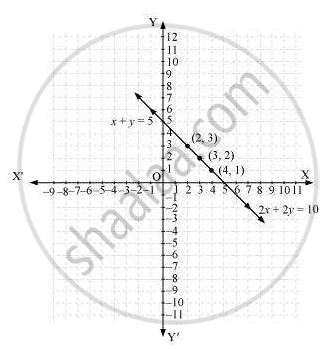# Which of the following pairs of linear equations are consistent/ inconsistent? If consistent, obtain the solution graphically: x + y = 5, 2x + 2y = 10 - Mathematics

Which of the following pairs of linear equations are consistent/ inconsistent? If consistent, obtain the solution graphically: x + y = 5, 2x + 2y = 10

#### Solution

x + y = 5

2x + 2y = 10

a_1/a_2=1/2, b_1/b_2=1/2, c_1/c_2 = 5/10 =1/2

Since a_1/a_2=b_1/b_2=c_1/c_2

Therefore, these linear equations are coincident pair of lines and thus have infinite number of possible solutions. Hence, the pair of linear equations is consistent.

x + y = 5

x = 5 - y

 x 4 3 2 y 1 2 3

And, 2x + 2y = 10

x =(10-2y)/2

 x 4 3 2 y 1 2 3

Hence, the graphic representation is as follows.From the figure, it can be observed that these lines are overlapping each other. Therefore, infinite solutions are possible for the given pair of equations

Concept: Graphical Method of Solution of a Pair of Linear Equations
Is there an error in this question or solution?

#### APPEARS IN

NCERT Class 10 Maths
Chapter 3 Pair of Linear Equations in Two Variables
Exercise 3.2 | Q 4.1 | Page 50